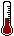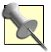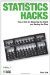# Hack 1. Know the Big SecretStatisticians know one secret thing that makes them seem smarter than everybody else.

The primary purpose of statistics as a scientific methodology is to make probability statements about samples of scores. Before we jump into that, we need some quick definitions to get us rolling, both to understand this hack and to lay a foundation for other statistics hacks.

Samples are numeric values that you have gathered together and can see in front of you that represent some larger population of scores that you have not gathered together and cannot see in front of you. Because these values are almost always numbers that indicate the presence or level of some characteristic, measurement folks call these values scores. A probability statement is a statement about the likelihood of some event occurring.

Probability is the heart and soul of statistics. A common perception of statisticians, in fact, is that they mainly calculate the exact likelihood that certain events of interest will occur, such as winning the lottery or being struck by lightning. Historically, the person who had the tools to calculate the likely outcome of a dice game was the same person who had the tools to describe a large group of people using only a few summary statistics.

So, traditionally, the teaching of statistics includes at least some time spent on the basic rules of probability: the methods for calculating the chances of various combinations or permutations of possible outcomes. More common applications of statistics, however, are the use of descriptive statistics to describe a group of scores, or the use of inferential statistics to make guesses about a population of scores using only the information contained in a sample of scores. In social science, the scores usually describe either people or something that is happening to them.

It turns out, then, that researchers and measurers (the people who are most likely to use statistics in the real world) are called upon to do more than calculate the probability of certain combinations and permutations of interest. They are able to apply a wide variety of statistical procedures to answer questions of varying levels of complexity without once needing to compute the odds of throwing a pair of six-sided dice and getting three 7s in a row.Those odds are .005 or 1/2 of 1 percent if you start from scratch. If you have already rolled two 7s, you have a 16.6 percent chance of rolling that third 7.

#### The Big Secret

The key reason that probability is so crucial to what statisticians do is because they like to make probability statements about the scores in real or theoretical distributions.A distribution of scores is a list of all the different values and, sometimes, how many of each value there are.

For example, if you know that a quiz just administered in a class you are taking resulted in a distribution of scores in which 25 percent of the class got 10 points, then I might say, without knowing you or anything about you, that there is a 25 percent chance that you got 10 points. I could also say that there is a 75 percent chance that you did not get 10 points. All I have done is taken known information about the distribution of some values and expressed that information as a statement of probability. This is a trick. It is the secret trick that all statisticians know. In fact, this is mostly all that statisticians ever do!

Statisticians take known information about the distribution of some values and express that information as a statement of probability. This is worth repeating (or, technically, threepeating, as I first said it five sentences ago). Statisticians take known information about the distribution of some values and express that information as a statement of probability.

Heavens to Betsy, we can all do that. How hard could it be? Imagine that there are three marbles in an otherwise empty coffee can. Further imagine that you know that only one of the marbles is blue. There are three values in the distribution: one blue marble and two marbles of some other color, for a total sample size of three. There is one blue marble out of three marbles. Oh, statistician, what are the chances that, without looking, I will draw the blue marble out first? One out of three. 1/3. 33 percent.

To be fair, the values and their distributions most commonly used by statisticians are a bit more abstract or complex than those of the marbles in a coffee can scenario, and so much of what statisticians do is not quite that transparent. Applied social science researchers usually produce values that represent the difference between the average scores of several groups of people, for example, or an index of the size of the relationship between two or more sets of scores. The underlying process is the same as that used with the coffee can example, though: reference the known distribution of the value of interest and make a statement of probability about that value.

The key, of course, is how one knows the distribution of all these exotic types of values that might interest a statistician. How can one know the distribution of average differences or the distribution of the size of a relationship between two sets of variables? Conveniently, past researchers and mathematicians have developed or discovered formulas and theorems and rules of thumb and philosophies and assumptions that provide us with the knowledge of the distributions of these complex values most often sought by researchers. The work has been done for us.

#### A Smaller, Dirtier Secret

Most of the procedures that statisticians use to take known information about a distribution of scores and express that information as a statement of probability have certain requirements that must be met for the probability statement to be accurate. One of these assumptions that almost always must be met is that the values in a sample have been randomly drawn from the distribution.

Notice that in the coffee can example I slipped in that "without looking" business. If some force other than random chance is guiding the sampling process, then the associated probabilities reported are simply wrong andhere's the worst partwe can't possibly know how wrong they are. Much, and maybe most, of the applied psychological and educational research that occurs today uses samples of people that were not randomly drawn from some population of interest.

College students taking an introductory psychology course make up the samples of much psychological research, for example, and students at elementary schools conveniently located near where an educational researcher lives are often chosen for study. This is a problem that social science researchers live with or ignore or worry about, but, nevertheless, it is a limitation of much social science research.Statistics Hacks: Tips & Tools for Measuring the World and Beating the Odds
ISBN: 0596101643
EAN: 2147483647
Year: 2004
Pages: 114
Authors: Bruce Frey

Similar book on Amazon